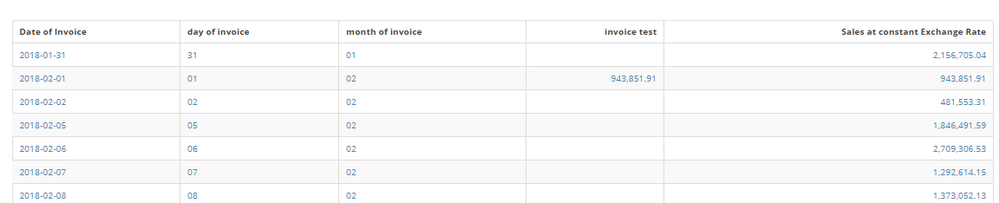# SUM of CASE in Beast Mode

Hi,

I am trying to sum a column based on date value output from another case statement, but i dont see anything getting calculated when i check the formula.

there are no formula validation issues.

`SUM((CASE when (CASE when DATE_FORMAT(`Date`,'%d')=1 	then 	(CASE when DATE_FORMAT(`Date`,'%m')=1 		then 'Open Month' else 			(CASE when DATE_FORMAT(`Date`,'%m')=4    				then 'Open Month' else    					(CASE when DATE_FORMAT(`Date`,'%m')=7        					then 'Open Month' else        						(CASE when DATE_FORMAT(`Date`,'%m')=10            						then 'Open Month' else '' end)					end)			end)	end)	else 	(CASE when LAST_DAY(`Date`)=`Date`     	then     	(CASE when DATE_FORMAT(`Date`,'%m')=6                    	then 'Close Month' else                    	(CASE when DATE_FORMAT(`Date`,'%m')=9                        	then 'Close Month' else                        	(CASE when DATE_FORMAT(`Date`,'%m')=12                            	then 'Close Month' else '' end)                         end)         end)     else     (CASE when CURRENT_DATE()=`Date`      	then 'Close Month' else '' end)     end)end)='Open Month' then `Total_HC_Opening` end))`

• You're just trying to sum up Total_HC_Opening for the first day of certain months?

I think you could really simplify this:

`SUM(CASE WHEN DATE_FORMAT(`Date`,'%d') = 1 AND DATE_FORMAT(`Date`,'%m') IN (1,4,7,10) THEN `Total_HC_Opening` END)`

Does this work?

Aaron
MajorDomo @ Merit Medical

**Say "Thanks" by clicking the heart in the post that helped you.
**Please mark the post that solves your problem by clicking on "Accept as Solution"
• When using DATE_FORMAT, the result is not a value, but a string.  You need to use single quotes and they need to be two characters long.  Something like this:

sum(case when DATE_FORMAT(`Date of Invoice`,'%d')='01' and DATE_FORMAT(`Date of Invoice`,'%m')='02' then `Sales at constant Exchange Rate` end)______________________________________________________________________________________________
“There is a superhero in all of us, we just need the courage to put on the cape.” -Superman
______________________________________________________________________________________________
• I had the same thought as @ST_-Superman-_ but it actually works for me as-is.

`SUM(CASE WHEN DATE_FORMAT(`Date`,'%d') = 1 AND DATE_FORMAT(`Date`,'%m') IN (1,4,7,10) THEN `Value1` END) `

See attached table sum file.

Also from this site the other attachment with format explanation.

Aaron
MajorDomo @ Merit Medical

**Say "Thanks" by clicking the heart in the post that helped you.
**Please mark the post that solves your problem by clicking on "Accept as Solution"

• Yes, I am trying the same as you are suggesting but still returns nothing.

• When i use only month from date format it is showing the calculation but when i add date as 1 it shows nothing, infact i tried with all possible date but comes up nothing.

• Spoiler

Hi All,

Thank you for your help, the code did not work initially because it was returning null value of day 1 of the month, but i had applied some more logic to it and now able to get the desiered output.